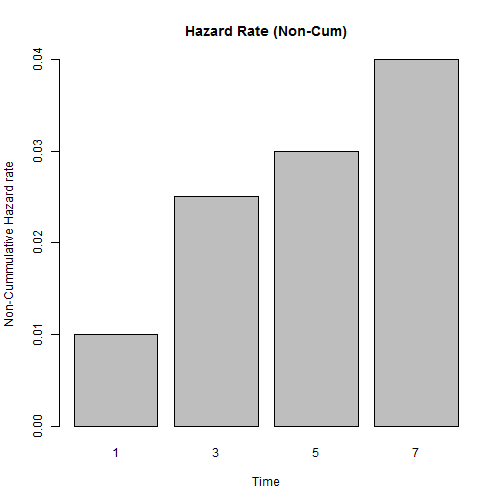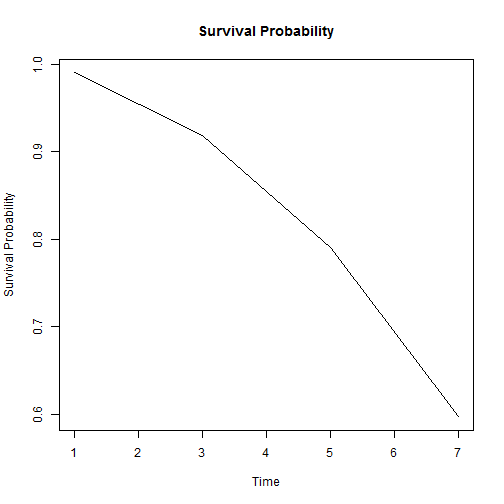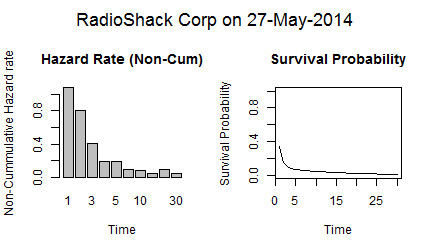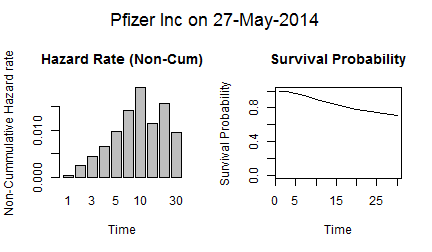We will first explain how credit curves are constructed (using a reduced-form model) before showing how the credule package can be used to build credit curves from the CDS quotes. We will look at 2 specific US Issuers as of 27 May 2014: Pfizer (Pfizer Inc - PFE) and Radioshak (RadioShack Corp - RSH).

## Credit Curve Bootstrapping Methodology

### Hazard rate and Survival Probability

The reduced-form model that we use here is based on the work of Jarrow and Turnbull (1995), who characterize a credit event as the first event of a Poisson counting process which occurs at some time $$t$$ with a probability defined as :

$$\text{Pr}\left[\tau the probability of a default occurring within the time interval [t,t+dt) conditional on surviving to time t, is proportional to some time dependent function \(\lambda(t)$$, known as the hazard rate, and the length of the time interval dt. We make the simplifying assumption that the hazard rate process is deterministic. By extension, this assumption also implies that the hazard rate is independent of interest rates and recovery rates. From this definition, we can calculate the continuous time survival probability to the time $$T$$ conditional on surviving to the valuation time $$t_{V}$$ by considering the limit $$\rightarrow0$$. It can be shown that the survival probability is given by:

$$Q(t_{V},T)=\exp\left(-\int_{t_{V}}^{T}\lambda(s)\, ds\right)$$

Finally, we assume that the hazard rate function is a step-wise constant function. In the below example, the hazard rate between time 0 and 1Y is $$h_{0,1}=1\%$$ and the hazard rate between between 1Y and 3Y is $$h_{1,3}=2.5\%$$. Therefore we have the 1Y survival probability $$Q_{0,1}=exp(-h_{0,1}\times1)=99\%$$ and the 3Y survival probability $$Q_{1,3}=Q_{0,1}*exp(-h_{1,3}\times2)=91.9\%$$.

tenor hazardrate survprob
1 1.00% 99.00%
3 2.50% 91.85%
5 3.00% 79.06%
7 4.00% 59.75%### Valuing the Premium Leg

The premium leg is the series of payments of the default swap spread made to maturity or to the time of the credit event, whichever occurs first. It also includes the payment of premium accrued from the previous premium payment date until the time of the credit event. Assume that there are $$n=1,…,N$$ contractual payment dates $$t_1,… ,t_N$$ where $$t_N$$ is the maturity date of the default swap.

The present value of the premium leg is given by:

$$\text{PL PV}(t_{V},t_{N})=S(t_{0},t_{N})\sum_{n=1}^{N}\Delta(t_{n-1},t_{n},B)Z(t_{V},t_{n})\left[Q(t_{V},t_{n})+\frac{1_{PA}}{2}(Q(t_{V},t_{n-1})-Q(t_{V},t_{n}))\right]$$

where:

• N is the total number of premiums. $$n=1,\ldots,N$$ is the index for these premiums; premium dates are denoted $$t_{1},\ldots,t_{N}$$. $$t_{N}$$ is the maturity date of the swap
• $$S(t_{0},t_{N})$$ is the spread of a Credit Default Swap that matures on $$t_{N}$$
• $$\Delta(t_{n-1},t_{n},B)$$ is the day count fraction between premium dates $$t_{n-1}$$ and $$t_{n}$$ in the appropriate basis convention denoted by B (ACT/360 in our model)
• $$Q(t_{V},t_{n})$$ is the arbitrage-free survival probability of the reference entity from valuation time $$t_{V}$$ to premium time $$t_{n}$$
• $$Z(t_{V},t_{n})$$ is the risk-free discount factor from valuation date to the premium date $$n$$
• $1_{PA}$equals 1 if premium accrued (PA) are included in the premium leg calculation, 0 otherwise.

### Valuing the Default Leg

The default leg (or protection leg) is the contingent payment of (100\% - R) on the face value of the protection made following the credit event. R is the expected recovery rate. In pricing the default leg, it is important to take into account the timing of the credit event because this can have a significant effect on the present value of the protection leg especially for longer maturity default swaps. Within the hazard rate approach we can solve this timing problem by conditioning on each small time interval $$[s,s+ds]$$ between time $$t_V$$ and time $$t_N$$ at which the credit event can occur. We calculate the expected present value of the recovery payment as:

$$\text{DL PV}(t_{V},t_{N})=(1-R)\int_{t_{V}}^{t_{N}}Z(t_{V},s)Q(t_{V},s)\lambda(s)ds$$

The integral makes this expression tedious to evaluate. It is possible to show that we can, without any material loss of accuracy, simply assume that the credit event can only occur on a finite number M of discrete points per year. In this case, the default leg value can be expressed as:

$$\text{DL PV}(t_{V},t_{N})=(1-R)\sum_{m=1}^{M\times t_{N}}Z(t_{V},t_{m})\left(Q(t_{V},t_{m-1})-Q(t_{V},t_{m})\right)$$

where:

• M is the number of discrete points per year on which we assume a credit event can happen. $$t_{N}$$ is the maturity of the swap. $$m=1,\ldots,M\times t_{N}$$ is the index the discrete point on which a credit event can happen between the valuation date and the maturity of the swap. We choose $$M=12$$ (monthly discretization) to keep the computation lightweight. D. O\'kane and S. Turnbull (2003) compare the results of a daily discretization ($$M=365$$) and a monthly discretization ($$M=12$$) and shows that monthly intervals has an absolute error which is well inside the bid-offer spread; hence monthly discretization can be used to keep the model fast and simple.
• $$R$$ is the recovery rate
• $$Q(t_{V},t_{m})$$ is the arbitrage-free survival probability of the reference entity from valuation time $$t_{V}$$ to premium time $$t_{m}$$
• $$Z(t_{V},t_{m})$$ is the risk-free discount factor from valuation date to the date m

### Calibration of the Credit Curve

Calibration of the model imply finding an hazard rate (non-cumulative hazard rate) function that matches the market CDS spreads. We make the following assumptions:

• Swap premium payments are made quarterly following a business day calendar
• Hazard rate is a piece-wise constant function of time (i.e. hazard rates are independent from interest rates)
• Recovery rate is constant

The construction of the hazard rate term structure is done by an iterative process called bootstrapping. Let's assume we have quotes for 1Y, 3Y, 5Y and 7Y for a given issuer. From the 1Y CDS spread $$s_{1Y}$$, we will find the hazard rate $$\lambda_{0,1}$$ which equates the present value of the premium leg and of the protection leg. From the 3Y spread $$s_{3Y}$$, we will find the hazard rate $$\lambda_{1,3}$$ which equates the present value of the premium leg to the present value of the protection leg. By iterating this process, we obtain the hazard rates: $$\lambda_{0,1},\lambda_{1,3},\lambda_{3,5},\lambda_{5,7}$$. We can also easily calculate the survival probabilities from this hazard rate term structure (as we have seen earlier).

## Market data

yieldcurveTenor = c(1.0,2.0,3.0,4.0,5.0,7.0,
10.0,15.0,20.0,30.0)
yieldcurveRate = c(0.002585,0.005034,0.008981,0.012954,0.016452,
0.021811,0.027007,0.031718,0.033834,0.035056)

1 2 3 4 5 7 10 15 20 30
0.26% 0.50% 0.90% 1.30% 1.65% 2.18% 2.70% 3.17% 3.38% 3.51%
cdsTenor = c(1,2,3,4,5,7,10,15,20,30)
cdsSpread_PFE = c(0.0003,0.0009,0.0015,0.0021,0.0028,0.0043,0.0061,0.0063, 0.0068,0.0066)

1 2 3 4 5 7 10 15 20 30
3 9 15 21 28 43 61 63 68 66
cdsTenor = c(1,2,3,4,5,7,10,15,20,30)
cdsSpread_RSH = c(0.6405,0.5956,0.5511,0.5144,0.4894,0.4511,0.4156,0.3815,0.3657,0.3506)

1 2 3 4 5 7 10 15 20 30
6405 5956 5511 5144 4894 4511 4156 3815 3657 3506

## Credit Curves

### RadioShack Corp, 27 May 2014

creditcurve_RSH = bootstrapCDS(yieldcurveTenor, yieldcurveRate,
cdsTenor, cdsSpread_RSH,
RR, premiumFrequency, defaultFrequency, accruedPremium)

tenor survprob hazrate
1 34.18% 107.37%
2 15.38% 79.84%
3 10.22% 40.91%
4 8.45% 18.95%
5 6.95% 19.61%
7 5.66% 10.22%
10 4.39% 8.51%
15 3.37% 5.29%
20 2.13% 9.16%
30 1.36% 4.48%### Pfizer Inc, 27 May 2014

creditcurve_PFE = bootstrapCDS(yieldcurveTenor, yieldcurveRate,
cdsTenor, cdsSpread_PFE,
RR, premiumFrequency, defaultFrequency, accruedPremium)

tenor survprob hazrate
1 99.95% 0.05%
2 99.70% 0.25%
3 99.25% 0.45%
4 98.59% 0.66%
5 97.65% 0.96%
7 94.92% 1.42%
10 89.69% 1.89%
15 84.71% 1.14%
20 78.37% 1.56%
30 71.27% 0.95%## Conclusion

It is interesting to compare the credit curve of these 2 issuers. A quick look at the 5Y spreads (market benchmark) shows that one issuer is much more risky than the other. Pfizer 5Y spread is at 28bp (i.e. 0.28\%), this is a pretty low CDS spreads (only 3bp more than the 5Y CDS on a sovereign like Finland, as of 3 August 2015). On the other hand, Radioshack 5Y spread is at 4894bp (i.e. 48.94\%) which is a very high CDS spread. As a comparison, it is more than two times than the Greece 5Y CDS as of 3 August 2015 (2203.70bp).

Futhermore, we can observe than the hazard rate does not have the same dynamic for both issuers. For Pfizer, the hazard rate curve is upward sloping (i.e hazard rate increase over time) whereas for Radioshack, the hazard rate curve is downward sloping. Downward sloping curve is commonly observed for stressed assets/speculative-grade firms (Radioshack rating is CCC as of 27 May 2014) and it translates the investors's expecation of a short term default. In other words, investors think that the issuer has room to improve with age (become less risky) or less potential to worsen considering that it is very risky today.

## References

• JP Morgan. Credit Derivatives: A Primer. JP Morgan Credit Derivatives and Quantitative Research (January 2005)
• D. O\'kane and S. Turnbull. Valuation of Credit Default Swaps. Lehman Brothers Quantitative Credit Research (Apr. 2003)
• Standard CDS Examples. Supporting document for the Implementation of the ISDA CDS Standard Model (Oct. 2012)